## AP State Syllabus SSC 10th Class Maths Solutions 5th Lesson Quadratic Equations Optional Exercise

AP State Board Syllabus AP SSC 10th Class Maths Textbook Solutions Chapter 5 Quadratic Equations Optional Exercise Textbook Questions and Answers.

### 10th Class Maths 5th Lesson Quadratic Equations Optional Exercise Textbook Questions and Answers

Question 1.
Some points are plotted on a plane. Each point is joined with all remaining points by line segments. Find the number of points if the number of line segments are 10.
Number of distinct line segments that can be formed out of n-points = $$\frac{\mathrm{n}(\mathrm{n}-1)}{2}$$
Given: No. of line segments
$$\frac{\mathrm{n}(\mathrm{n}-1)}{2}$$ = 10
⇒ n2 – n = 20
⇒ n2 – n – 20 = 0
⇒ n2 – 5n + 4n – 20 = 0
⇒ n(n – 5) + 4(n – 5) = 0
⇒ (n – 5) (n + 4) = 0
⇒ n – 5 = 0 (or) n + 4 = 0
⇒ n = 5 (or) -4
∴ n = 5 [n – can’t be negative]Question 2.
A two digit number is such that the product of its digits, is 8. When 18 is added to the number, they interchange their places. Determine the number.
Let the digit in the units place = x
Let the digit in the tens place = y
∴ The number = 10y + x
By interchanging the digits the number becomes 10x + y
By problem (10x + y) – (10y + x) = 18
⇒ 9x – 9y = 18
⇒ 9(x – y) =18
⇒ x – y = $$\frac{18}{9}$$ = 2
⇒ y = x – 2
(i.e.) digit in the tens place = x – 2
digit in the units place = x
Product of the digits = (x – 2) x
By problem x2 – 2x = 8
x2 – 2x – 8 = 0
⇒ x2 – 4x + 2x – 8 = 0
⇒ x(x – 4) + 2(x – 4) = 0
⇒ (x – 4) (x + 2) = 0
⇒ x – 4 = 0 (or) x + 2 = 0
⇒ x = 4 (or) x = -2
∴ x = 4 [∵ x can’t be negative]
∴ The number is 24.Question 3.
A piece of wire 8m in length is cut into twp pieces and each piece is bent into a square. Where should the cut in the wire be made if the sum of the areas of these squares is to be 2 m2?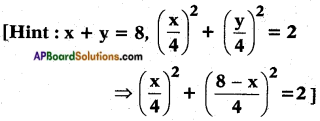Let the length of the first peice = x m
Then length of the second piece = 8 – x m
∴ Side of the 1st square = $$\frac{x}{4}$$ m and
Side of the second square = $$\frac{8-x}{4}$$ m
sum of the areas = 2 m2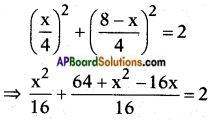⇒ x2 + 64 + x2 – 16x = 16 × 2 = 32
⇒ 2x2 – 16x + 64 = 32
⇒ 2x2 – 16x + 32 = 0
⇒ 2(x2 – 8x + 16)= 0
⇒ x2 – 8x + 16 = 0
⇒ x2 – 4x – 4x + 16 = 0
⇒ x(x – 4) – 4(x – 4) = 0
⇒ (x – 4) (x – 4) = 0
∴ x = 4
∴ The cut should be made at the centre making two equal pieces of length 4 m, 4 m.

Question 4.
Vinay and Praveen working together can paint the exterior of a house in 6 days. Vinay by himself can complete the job in 5 days less than Praveen. How long will it take Vinay to complete the job by himself?
Let the time taken by Vinay to complete the job = x days
Then the time taken by Praveen to complete the job = x + 5 days
Both worked for 6 days to complete a job.
∴ Total Work done by them is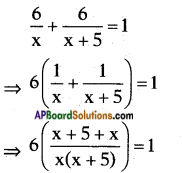⇒ 6(2x + 5) = x2 + 5x
⇒ x2 – 7x – 30 = 0
⇒ x2 – 10x + 3x – 30 = 0
⇒ x(x – 10) + 3(x – 10) = 0
⇒ (x – 10) (x + 3) = 0
⇒ x – 10 = 0 (or) x + 3 = 0
⇒ x = 10 (or) x = -3
∴ x = 10 (∵ x can’t be negative)
∴ Time taken by Vinay = x = 10 days
Time taken by Praveen = x + 5 = 15 days.Question 5.
Show that the sum of the roots of a quadratic equation ax2 + bx + c = 0 is $$\frac{-b}{a}$$.
Let the Q.E. = ax2 + bx + c = 0 (a ≠ 0)
⇒ ax2 + bx = -c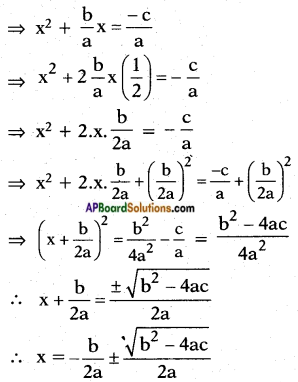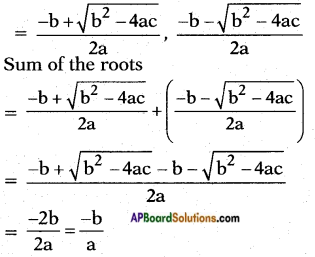∴ Sum of roots of a Q.E. is $$\frac{-b}{a}$$

Question 6.
Show that the product of the roots of a quadratic equation ax2 + bx + c = 0 is $$\frac{c}{a}$$.
Let the Q.E. = ax2 + bx + c = 0 (a ≠ 0)
⇒ ax2 + bx = -c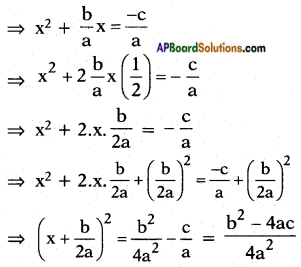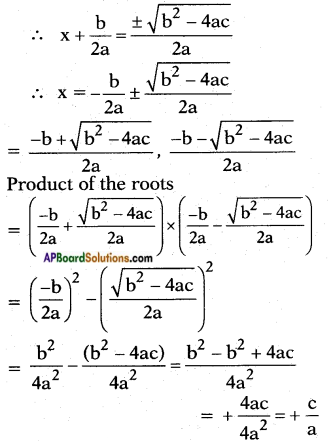Question 7.
The denominator of a fraction is one more than twice the numerator. If the sum of the fraction and its reciprocal is 2$$\frac{16}{21}$$ find the fraction.
Let the numerator = x
then denominator = 2x + 1
Then the fraction = $$\frac{x}{2x+1}$$
Its reciprocal = $$\frac{2x+1}{x}$$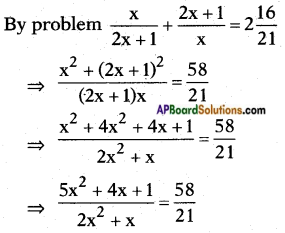105x2 + 84x + 21 = 116x2 + 58x
11x2 – 26x – 21 = 0
11x2 – 33x + 7x – 21 = 0
11x (x – 3) + 7 (x – 3) = 0
(x – 3) (11x + 7) = 0
⇒ x – 3 = 0 (or) 11x + 7 = 0
⇒ x = 3 (or) $$\frac{-7}{11}$$
∴ x = 3
Numerator = 3;
Denominator = 2 × 3 + 1 = 7
Fraction = $$\frac{3}{7}$$.

Question 8.
A ball is thrown vertically upwards from the top of a building of height 29.4m and with an initial velocity 24.5m/sec. If the height H of the ball from the ground level is given by H = 29.4 + 24.5t – 4.9t2, then find the time taken by the ball to reach the ground.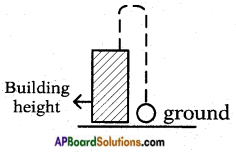Initial velocity ‘U’ = 24.5
height of the ball from the ground can be expressed as
H = 29.4 + 24.5 t – 4.9 t2
The ball has to reach the ground in ‘t’ seconds, which means Height from ground H = 0
So 29.4 + 24.5t – 4.9t2 = 0 = H
⇒ 4.9 t2 – 24.5t – 29.4 = 0
⇒ 4.9 [t2 – 5t – 6] = 0
∴ t2 – 5t – 6 = 0
⇒ t2 – 6t + t – 6 = 0
⇒ t(t – 6) + 1 (t – 6) = 0
(t – 6) (t + 1) = 0
⇒ t – 6 = 0
∴ t = 6 or t + 1 = 0
⇒ t = -1 but ‘t’ cannot be negative
So t = 6
it means in 6 seconds of time the ball reaches ground.